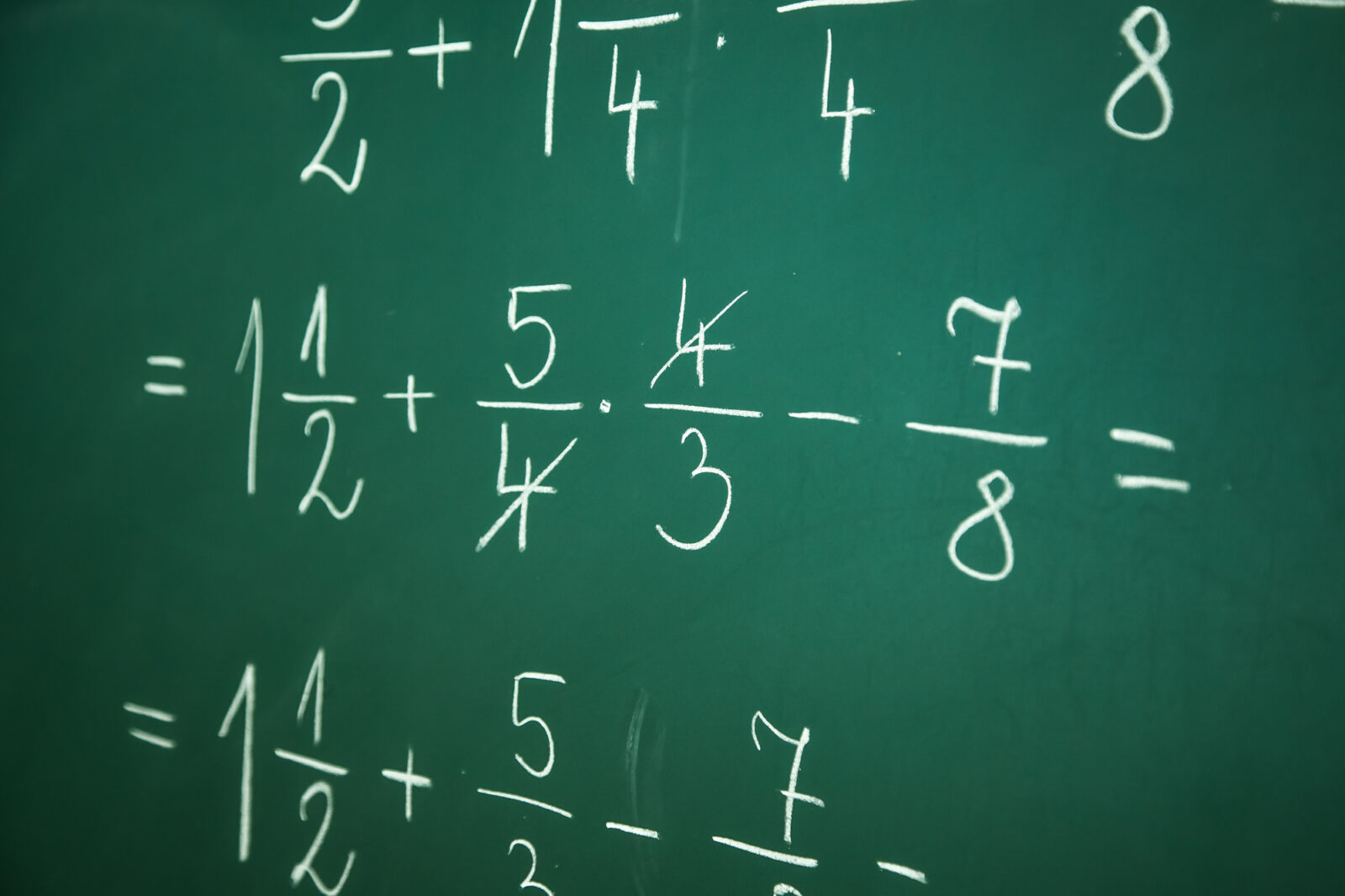# How to: Adding, Subtracting, Multiplying, and Dividing FractionsWhat do we use in everyday life that we don’t even realize is a math skill? Fractions! Have you ever had to convert a recipe to a smaller or bigger serving?

What about how many yards someone runs in football? They ran 20 out of the 100 yards. Yes, that’s a fraction too.

But what would you do if someone told you to sit down and work on fractions right now? You probably wouldn’t know where to start adding subtracting multiplying and dividing fractions, right?

Whether you never learned it, weren’t paying attention when you did, or just need a refresher – we’ve got your guide.

And if you read all this just to decide no, I’m not willing to do the math, we’ve got your link to a fractions calculator too!

## Fraction Reminders

When you’re dealing with adding subtracting multiplying and dividing fractions, there are few things you want to keep in mind.

One, it’s always better to reduce. Reducing fractions means seeing how many times the top number (numerator) goes into the denominator (bottom number).

For example, if you got the answer of 5 over 25 (5/25) and you did the math correctly, it’s still wrong. The correct answer, reduced down, is 1/5.

Why? Because 5 (the numerator) goes into the denominator (25) five times. So we reduce and get 1/5 from 5/25.

Second, when you’re doing anything with fractions that involve two sets always keep your work organized. With that, you’re ready for our how-to guide below.

The process for adding and subtracting fractions is the same, save for the mathematical function. If both bottom numbers are the same, you’re in luck and can get through the problem quickly.

For equations with the same denominator, all you have to do is add the numerators.

Example: 1/4+2/4= 3/4. If you learn better in words, that same equation looks like this.

Someone divides a pie into four slices onto different plates. (Four is the denominator). There’s one plate with one slice of pie (1/4) and one plate with two slices of pie (2/4).

If I tried to put them back in the pie dish, how much pie would I have left? Well, we put the one slice (out of the original four) in to represent 1/4.

Then we put the other two slices of the original pie in – so now there are three pieces of the original four in the pan.

## Subtracting Fractions

To keep from having too many numbers, let’s stay with our pie example. The idea of subtracting fractions is the same- only worry about the numerators when the denominators are equal.

So, if someone took one slice out of the three slices we had in the pie tin from the previous question out, how many are left?

3/4 – 1/4 = 2/4. Is that our final answer?

No, if you caught the trick, 2/4 is too big of a number because we can reduce it down. Two goes into four twice, so the actual answer is 1/2.

We have 1/2 of the pie left, which is the same thing as 2/4.

## Common Denominators

When things get tricky is when you don’t have the same bottom numbers. When that happens, we have to make the denominators the same, by multiplying one set to match the number in the other.

Let’s say we have an equation that asks us to add 1/3 and 1/6. We can’t just add through and get 2/9. That’s incorrect. We need to make three matches the six.

Thankfully, we picked easy numbers and know that 3 goes into six twice.

So, we look at 1/3 and multiply BOTH TOP AND BOTTOM by two. Now we have 2/6 instead of 1/3.

The new equation is 2/6 + 1/6 which equals 3/6. Reduce it down to 1/2 and you get your final answer.

### Subtracting

The exact same is true for subtraction. If we have 1/3 – 1/6 we do all the exact steps as above. We multiply 1/3 by two and get 2/6 – then, instead of adding, we subtract.

So, 2/6 – 1/6 = 1/6 and that’s as low as our fraction can go – so there’s no need to reduce.

## Multiplying Fractions

Multiplying fractions is almost easier than adding and subtracting them because you don’t need to find a common denominator.

To multiply two fractions, you multiply across. Let’s list the steps, then do an example.

1. Reduce fractions if needed
2. Set up equation
3. Multiply across

Example: Multiply the fractions 2/10 and 2/3

1. Reduce the fraction 2/10 to 1/5.
2. Now our equation is 1/5 X 2/3
3. Multiply the top numbers (1 x 2 =2) and the bottom (5 x 3 = 15)

The answer you get is 2/15. Two doesn’t go into 15 evenly, so we can’t reduce.

## Dividing Fractions

Ok, hang on to your hat here. We promise that dividing fractions isn’t hard – but there are two extra steps you have to remember.

Instead of dividing the numbers, you’re going to multiply. Let’s talk through how a division problem becomes multiplication.

The way to remember and work through this problem is to leave, switch, flip.

If you have 1/3 divided by 2/5 you’re eventually going to get 5/6. Here’s what we did.

1. Leave 1/3 alone. That’s the leave of leave, switch, flip.
2. Switch the division sign to multiplication.
3. FLIP the second fraction upside down. That means 2/5 becomes 5/2.

Now that you have 1/3 x 5/2 you can do the multiplication process from above. 1 x 5 = 5 and 2 x 3 = 6.